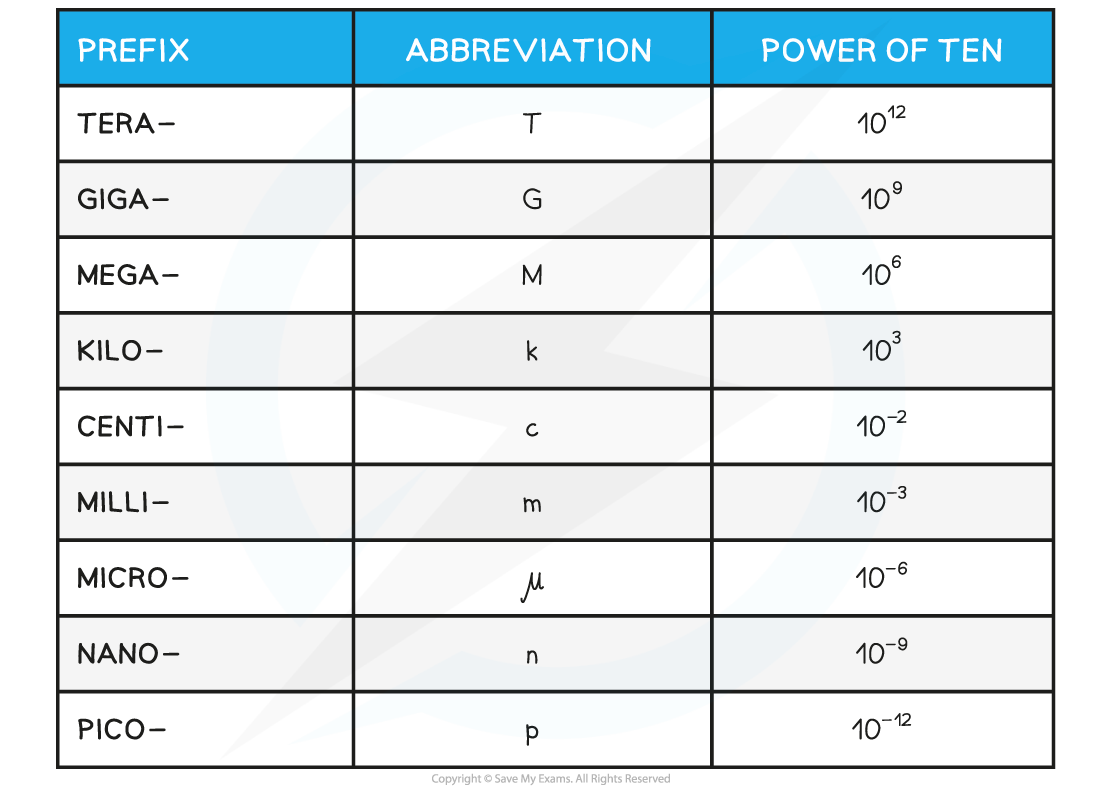# IB DP Physics: SL复习笔记1.1.2 Using Scientific Notation

### Scientific Notation & Metric Multipliers

#### Scientific Notation

• In physics, measured quantities cover a large range from the very large to the very small
• Scientific notation is a form that is based on powers of 10
• The scientific form must have one digit in front of the decimal place
• Any remaining digits remain behind the decimal place
• The magnitude of the value comes from multiplying by 10n where n is called 'the power'
• This power is positive when representing large numbers or negative when representing small numbers

#### Worked Example

Express 4 600 000 in scientific notation.

Step 1: Write the convention for scientific notation

• To convert into scientific notation, only one digit may remain in front of the decimal point
• Therefore, the scientific notation must be 4.6 × 10n
• The value of n is determined by the number of decimal places that must be moved to return to the original number (i.e. 4 600 000)

Step 2: Identify the number of digits after the 4

• In this case, that number is +6

Step 3: Write the final answer in scientific notation

• The solution is: 4.6 × 106

#### Metric Multipliers

• When dealing with magnitudes of 10, there are metric names for many common quantities
• These are known as metric multipliers and they change the size of the quantity they are applied to
• They are represented by prefixes that go in front of the measurement
• Some common examples that are well-known include
• kilometres, km (× 103)
• centimetres, cm (× 10–2)
• milligrams, mg (× 103)
• Metric multipliers are represented by a single letter symbol such as centi- (c) or Giga- (G)
• These letters go in front of the quantity of interest
• For example, centimetres (cm) or Gigawatts (GW)

Common Metric Multipliers Table#### Worked Example

What is the answer to the addition of 3.6 Mm + 2700 km in metres?

Step 1: Check which metric multipliers are in this problem

• M represents Mega- which is × 106 (not milli- which is small m!)
• k represents kilo- which is a multiplier of × 103

Step 2: Apply these multipliers to get both quantities to be metres

3.6 × 106 m  +  2.7 × 106 m

Step 3: Write the final answer in units of metres

6.3 × 106 m

#### Exam Tip

You are expected to know metric multipliers for your exams. Make sure you become familiar with them in order to avoid any mistakes.

### Significant Figures

• Significant figures are the digits that accurately represent a given quantity
• Significant figures describe the precision with which a quantity is known
• If a quantity has more significant figures then more precise information is known about that quantity

#### Rules for Significant Figures

• Not all digits that a number may show are significant
• In order to know how many digits in a quantity are significant, these rules can be followed
• Rule 1: In an integer, all digits count as significant if the last digit is non-zero
• Example: 702 has 3 significant figures
• Rule 2: Zeros at the end of an integer do not count as significant
• Example: 705,000 has 3 significant figures
• Rule 3: Zeros in front of an integer do not count as significant
• Example: 0.002309 has 4 significant figures
• Rule 4: Zeros at the end of a number less than zero count as significant, but those in front do not.
• Example: 0.0020300 has 5 significant figures
• Rule 5: Zeros after a decimal point are also significant figures.
• Example: 70.0 has 3 significant figures
• Combinations of numbers must always be to the smallest number significant figures

#### Worked Example

What is the solution to this problem to the correct number of significant figures: 18 × 384?

Step 1: Identify the smallest number of significant figures

• 18 has only 2 significant figures, while 384 has 3 significant figures
• Therefore, the final answer should be to 2 significant figures

Step 2: Do the calculation with the maximum number of digits

18 × 384 = 6912

Step 3: Round to the final answer to 2 significant figures

6.9 × 103